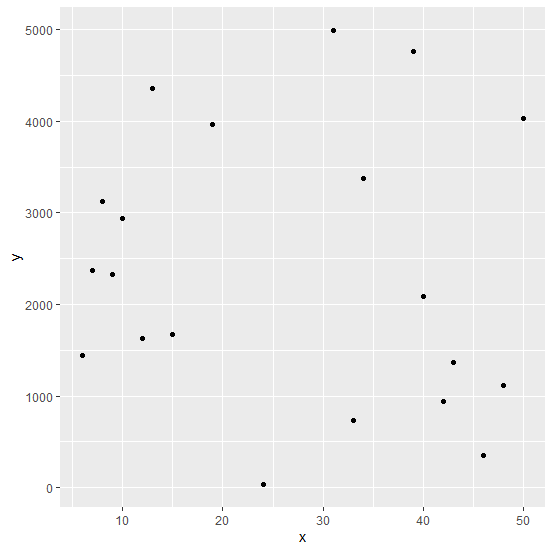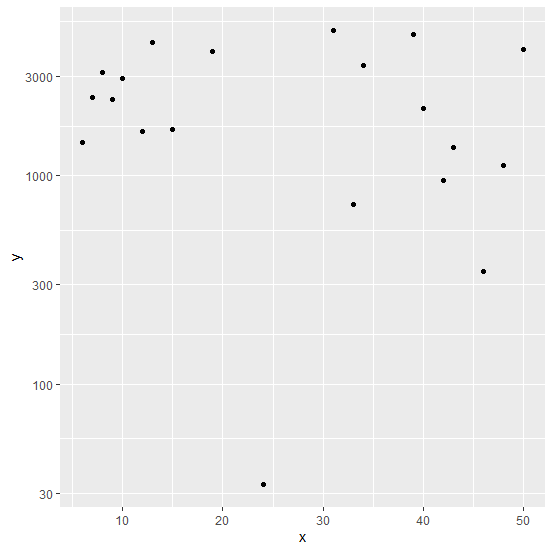# How to create a scatterplot with log10 of dependent variable in R?

Most of the times, the relationship between independent variable and dependent variable is not linear. Therefore, we want to transform the dependent variable or independent variable based on our experiences. Hence, we also want to plot those transformations to visualize the relationship, one such transformation is taking log10 of the dependent variable. To plot this transformation of the dependent variable, we can use scale_y_continuous(trans='log10').

## Example

Consider the below data frame −

set.seed(10)
x <-sample(1:50,20)
y <-sample(1:5000,20)
df <-data.frame(x,y)

Creating a scatterplot between x and y −

## Example

library(ggplot2)
ggplot(df,aes(x,y))+geom_point()

## OutputCreating a scatterplot between x and log10 of y &minus

ggplot(df,aes(x,y))+geom_point()+scale_y_continuous(trans='log10')

## Output